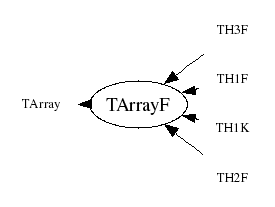library: libCore#include "TArrayF.h"

# TArrayF

class description - header file - source file

## class TArrayF: public TArray### Function Members (Methods)

Display options:
Show inherited
Show non-public
public:
 TArrayF() TArrayF(Int_t n) TArrayF(const TArrayF& array) TArrayF(Int_t n, const Float_t* array) virtual ~TArrayF() void AddAt(Float_t c, Int_t i) void Adopt(Int_t n, Float_t* array) Float_t At(Int_t i) const static TClass* Class() void Copy(TArrayF& array) const const Float_t* GetArray() const Float_t* GetArray() Int_t TArray::GetSize() const Stat_t GetSum() const virtual TClass* IsA() const TArrayF& operator=(const TArrayF& rhs) Float_t& operator[](Int_t i) Float_t operator[](Int_t i) const static TArray* TArray::ReadArray(TBuffer& b, const TClass* clReq) void Reset() void Reset(Float_t val) virtual void Set(Int_t n) void Set(Int_t n, const Float_t* array) virtual void ShowMembers(TMemberInspector& insp, char* parent) virtual void Streamer(TBuffer& b) void StreamerNVirtual(TBuffer& b) static void TArray::WriteArray(TBuffer& b, const TArray* a)
protected:
 Bool_t TArray::BoundsOk(const char* where, Int_t at) const Bool_t TArray::OutOfBoundsError(const char* where, Int_t i) const

### Data Members

public:
 Float_t* fArray [fN] Array of fN floats Int_t TArray::fN Number of array elements

## Class Description

```
TArrayF

Array of floats (32 bits per element).

```
TArrayF()
``` Default TArrayF ctor.
```
TArrayF(Int_t n)
``` Create TArrayF object and set array size to n floats.
```
TArrayF(Int_t n, const Float_t *array)
``` Create TArrayF object and initialize it with values of array.
```
TArrayF(const TArrayF &array)
``` Copy constructor.
```
TArrayF & operator=(const TArrayF &rhs)
``` TArrayF assignment operator.
```
~TArrayF()
``` Delete TArrayF object.
```
``` Adopt array arr into TArrayF, i.e. don't copy arr but use it directly
in TArrayF. User may not delete arr, TArrayF dtor will do it.
```
``` Add float c at position i. Check for out of bounds.
```
void Set(Int_t n)
``` Set size of this array to n floats.
A new array is created, the old contents copied to the new array,
then the old array is deleted.
This function should not be called if the array was declared via Adopt.
```
void Set(Int_t n, const Float_t *array)
``` Set size of this array to n floats and set the contents.
This function should not be called if the array was declared via Adopt.
```
void Streamer(TBuffer &b)
``` Stream a TArrayF object.
```

void Copy(TArrayF &array)
`{array.Set(fN,fArray);}`
const Float_t * GetArray()
`{ return fArray; }`

`{ return fArray; }`

`{Stat_t sum=0; for (Int_t i=0;i<fN;i++) sum+=fArray[i]; return sum;}`
void Reset()
`{memset(fArray, 0, fN*sizeof(Float_t));}`
void Reset(Float_t val)
`{for (Int_t i=0;i<fN;i++) fArray[i] = val;}`

Author: Rene Brun 06/03/95
Last update: root/cont:\$Name: \$:\$Id: TArrayF.cxx,v 1.14 2005/11/16 20:07:50 pcanal Exp \$